xHalf-lifeEncyclopedia
Number of
half-lives
elapsed
Fraction
remaining
| Percentage
remaining
0 1/1 100
1 1/2 50
2 1/4 25
3 1/8 12 .5
4 1/16 6 .25
5 1/32 3 .125
6 1/64 1 .563
7 1/128 0 .781
... ... ...
n 1/(2n) 100/(2n)

Half-life, abbreviated t½, is the period of time it takes for the amount of a substance undergoing decay to decrease by half. The name was originally used to describe a characteristic of unstable atoms (radioactive decay
Radioactive decay is the process by which an atomic nucleus of an unstable atom loses energy by emitting ionizing particles . The emission is spontaneous, in that the atom decays without any physical interaction with another particle from outside the atom...

), but it may apply to any quantity which follows a set-rate decay.

The original term, dating to 1907, was "half-life period", which was later shortened to "half-life" in the early 1950s.

Half-lives are used to describe quantities undergoing exponential decay—for example, radioactive decay—where the half-life is constant over the whole life of the decay, and is a characteristic unit (a natural unit of scale) for the exponential decay equation. However, a half-life can also be defined for non-exponential decay processes, although in these cases the half-life varies throughout the decay process. For a general introduction and description of exponential decay, see the article exponential decay. For a general introduction and description of non-exponential decay, see the article rate law.
Corresponding to sediments in environmental processes, if the half-life is greater than the residence time, then the radioactive nuclide will have enough time to significantly alter the concentration.
The converse of half-life is doubling time
Doubling time
The doubling time is the period of time required for a quantity to double in size or value. It is applied to population growth, inflation, resource extraction, consumption of goods, compound interest, the volume of malignant tumours, and many other things which tend to grow over time...

.

The table on the right shows the reduction of a quantity in terms of the number of half-lives elapsed.

## Probabilistic nature of half-life

A half-life describes the decay of discrete entities, such as radioactive atoms. In that case, it does not work to use the definition "half-life is the time required for exactly half of the entities to decay". For example, if there is just one radioactive atom with a half-life of 1 second, there will not be "half of an atom" left after 1 second. There will be either zero atoms left or one atom left, depending on whether or not the atom happens to decay.

Instead, the half-life is defined in terms of probability
Probability
Probability is ordinarily used to describe an attitude of mind towards some proposition of whose truth we arenot certain. The proposition of interest is usually of the form "Will a specific event occur?" The attitude of mind is of the form "How certain are we that the event will occur?" The...

. It is the time when the expected value
Expected value
In probability theory, the expected value of a random variable is the weighted average of all possible values that this random variable can take on...

of the number of entities that have decayed is equal to half the original number. For example, one can start with a single radioactive atom, wait its half-life, and measure whether or not it decays in that period of time. Perhaps it will and perhaps it will not. But if this experiment is repeated again and again, it will be seen that - on average - it decays within the half-life 50% of the time.

In some experiments (such as the synthesis of a superheavy element), there is in fact only one radioactive atom produced at a time, with its lifetime individually measured. In this case, statistical analysis is required to infer the half-life. In other cases, a very large number of identical radioactive atoms decay in the time-range measured. In this case, the law of large numbers
Law of large numbers
In probability theory, the law of large numbers is a theorem that describes the result of performing the same experiment a large number of times...

ensures that the number of atoms that actually decay is essentially equal to the number of atoms that are expected to decay. In other words, with a large enough number of decaying atoms, the probabilistic aspects of the process can be ignored.

There are various simple exercises that demonstrate probabilistic decay, for example involving flipping coins or running a computer program. For example, the image on the right is a simulation of many identical atoms undergoing radioactive decay. Note that after one half-life there are not exactly one-half of the atoms remaining, only approximately, due to random variation in the process. However, with more atoms (right boxes), the overall decay is smoother and less random than with fewer atoms (left boxes), in accordance with the law of large numbers
Law of large numbers
In probability theory, the law of large numbers is a theorem that describes the result of performing the same experiment a large number of times...

.

## Formulas for half-life in exponential decay

An exponential decay process can be described by any of the following three equivalent formulas: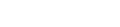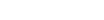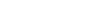where
•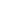is the initial quantity of the substance that will decay (this quantity may be measured in grams, moles, number of atoms, etc.),
•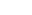is the quantity that still remains and has not yet decayed after a time t,
•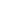is the half-life of the decaying quantity,
•Tau
Tau is the 19th letter of the Greek alphabet. In the system of Greek numerals it has a value of 300.The name in English is pronounced , but in modern Greek it is...

is a positive number called the mean lifetime of the decaying quantity,
• λ is a positive number called the decay constant of the decaying quantity.

The three parameters,, and λ are all directly related in the following way: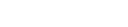where ln(2) is the natural logarithm
Natural logarithm
The natural logarithm is the logarithm to the base e, where e is an irrational and transcendental constant approximately equal to 2.718281828...

of 2 (approximately 0.693).

By plugging in and manipulating these relationships, we get all of the following equivalent descriptions of exponential decay, in terms of the half-life: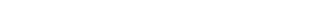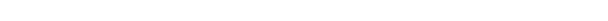Regardless of how it's written, we can plug into the formula to get
•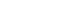as expected (this is the definition of "initial quantity")
•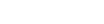as expected (this is the definition of half-life)
•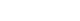, i.e. amount approaches zero as t approaches infinity
Limit of a function
In mathematics, the limit of a function is a fundamental concept in calculus and analysis concerning the behavior of that function near a particular input....

as expected (the longer we wait, the less remains).

### Decay by two or more processes

Some quantities decay by two exponential-decay processes simultaneously. In this case, the actual half-life T1/2 can be related to the half-lives t1 and t2 that the quantity would have if each of the decay processes acted in isolation: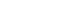For three or more processes, the analogous formula is: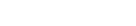For a proof of these formulas, see Decay by two or more processes.

### Examples

There is a half-life describing any exponential-decay process. For example:
• The current flowing through an RC circuit
RC circuit
A resistor–capacitor circuit ', or RC filter or RC network, is an electric circuit composed of resistors and capacitors driven by a voltage or current source...

or RL circuit
RL circuit
A resistor-inductor circuit ', or RL filter or RL network, is one of the simplest analogue infinite impulse response electronic filters. It consists of a resistor and an inductor, either in series or in parallel, driven by a voltage source.-Introduction:The fundamental passive linear circuit...

decays with a half-life of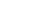or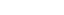, respectively.
• In a first-order chemical reaction
Chemical reaction
A chemical reaction is a process that leads to the transformation of one set of chemical substances to another. Chemical reactions can be either spontaneous, requiring no input of energy, or non-spontaneous, typically following the input of some type of energy, such as heat, light or electricity...

, the half-life of the reactant is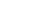, where λ is the reaction rate constant.
Radioactive decay is the process by which an atomic nucleus of an unstable atom loses energy by emitting ionizing particles . The emission is spontaneous, in that the atom decays without any physical interaction with another particle from outside the atom...

, the half-life is the length of time after which there is a 50% chance that an atom will have undergone nuclear
Atomic nucleus
The nucleus is the very dense region consisting of protons and neutrons at the center of an atom. It was discovered in 1911, as a result of Ernest Rutherford's interpretation of the famous 1909 Rutherford experiment performed by Hans Geiger and Ernest Marsden, under the direction of Rutherford. The...

decay. It varies depending on the atom type and isotope
Isotope
Isotopes are variants of atoms of a particular chemical element, which have differing numbers of neutrons. Atoms of a particular element by definition must contain the same number of protons but may have a distinct number of neutrons which differs from atom to atom, without changing the designation...

, and is usually determined experimentally.

## Half-life in non-exponential decay

The decay of many physical quantities is not exponential—for example, the evaporation of water from a puddle, or (often) the chemical reaction of a molecule. In such cases, the half-life is defined the same way as before: as the time elapsed before half of the original quantity has decayed. However, unlike in an exponential decay, the half-life depends on the initial quantity, and the prospective half-life will change over time as the quantity decays.

As an example, the radioactive decay of carbon-14
Carbon-14
Carbon-14, 14C, or radiocarbon, is a radioactive isotope of carbon with a nucleus containing 6 protons and 8 neutrons. Its presence in organic materials is the basis of the radiocarbon dating method pioneered by Willard Libby and colleagues , to date archaeological, geological, and hydrogeological...

is exponential with a half-life of 5730 years. A quantity of carbon-14 will decay to half of its original amount after 5730 years, regardless of how big or small the original quantity was. After another 5730 years, one-quarter of the original will remain. On the other hand, the time it will take a puddle to half-evaporate depends on how deep the puddle is. Perhaps a puddle of a certain size will evaporate down to half its original volume in one day. But on the second day, there is no reason to expect that one-quarter of the puddle will remain; in fact, it will probably be much less than that. This is an example where the half-life reduces as time goes on. (In other non-exponential decays, it can increase instead.)

The decay of a mixture of two or more materials which each decay exponentially, but with different half-lives, is not exponential. Mathematically, the sum of two exponential functions is not a single exponential function. A common example of such a situation is the waste of nuclear power stations, which is a mix of substances with vastly different half-lives. Consider a sample containing a rapidly decaying element A, with a half-life of 1 second, and a slowly decaying element B, with a half-life of one year. After a few seconds, almost all atoms of the element A have decayed after repeated halving of the initial total number of atoms; but very few of the atoms of element B will have decayed yet as only a tiny fraction of a half-life has elapsed. Thus, the mixture taken as a whole does not decay by halves.

## Half-life in biology and pharmacology

A biological half-life
Biological half-life
The biological half-life or elimination half-life of a substance is the time it takes for a substance to lose half of its pharmacologic, physiologic, or radiologic activity, as per the MeSH definition...

or elimination half-life is the time it takes for a substance (drug, radioactive nuclide, or other) to lose half of its pharmacologic, physiologic, or radiological activity. In a medical context, half-life may also describe the time it takes for the blood plasma
Blood plasma
Blood plasma is the straw-colored liquid component of blood in which the blood cells in whole blood are normally suspended. It makes up about 55% of the total blood volume. It is the intravascular fluid part of extracellular fluid...

concentration of a substance to halve ("plasma half-life") its steady-state. The relationship between the biological and plasma half-lives of a substance can be complex, due to factors including accumulation in tissues
Tissue (biology)
Tissue is a cellular organizational level intermediate between cells and a complete organism. A tissue is an ensemble of cells, not necessarily identical, but from the same origin, that together carry out a specific function. These are called tissues because of their identical functioning...

, active metabolite
Metabolite
Metabolites are the intermediates and products of metabolism. The term metabolite is usually restricted to small molecules. A primary metabolite is directly involved in normal growth, development, and reproduction. Alcohol is an example of a primary metabolite produced in large-scale by industrial...

s, and receptor
Receptor (biochemistry)
In biochemistry, a receptor is a molecule found on the surface of a cell, which receives specific chemical signals from neighbouring cells or the wider environment within an organism...

interactions.

While a radioactive isotope decays perfectly according to first order kinetics where the rate constant is fixed, the elimination of a substance from a living organism follows more complex kinetics.

For example, the biological half-life of water in a human is about 7 to 14 days, though this can be altered by behavior. The biological half-life of caesium
Caesium
Caesium or cesium is the chemical element with the symbol Cs and atomic number 55. It is a soft, silvery-gold alkali metal with a melting point of 28 °C , which makes it one of only five elemental metals that are liquid at room temperature...

in humans is between one and four months. This can be shortened by feeding the person Prussian blue
Prussian blue
Prussian blue is a dark blue pigment with the idealized formula Fe718. Another name for the color Prussian blue is Berlin blue or, in painting, Parisian blue. Turnbull's blue is the same substance but is made from different reagents....

, which acts as a solid ion exchange
Ion exchange
Ion exchange is an exchange of ions between two electrolytes or between an electrolyte solution and a complex. In most cases the term is used to denote the processes of purification, separation, and decontamination of aqueous and other ion-containing solutions with solid polymeric or mineralic 'ion...

r which absorbs the caesium while releasing potassium
Potassium
Potassium is the chemical element with the symbol K and atomic number 19. Elemental potassium is a soft silvery-white alkali metal that oxidizes rapidly in air and is very reactive with water, generating sufficient heat to ignite the hydrogen emitted in the reaction.Potassium and sodium are...

ions.